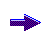Office Applications and Entertainment, Magic SquaresIndex About the Author

 16.3   Prime Numbers, Sum of Squares 16.3.1 Magic Squares (4 x 4) In another article Christian Boyer mentions an interesting method to construct Prime Number Magic Squares, using sums of squares, as published by André Gérardin early 1916 (Sphinx-Oedipe). The elements of two latin squares A1 (MC = 50) and B1 (MC = 120), with latin main diagoanls, result in a Prime Number Magic Square C (MC = 7588) with elements ci = ai2 + bi2, i = 1 ... 16 (ref. Attachment 16.3.1). The key to possible solutions is to find Correlated Magic Lines {ai, i = 1 ... 4} and {bj, j = 1 ... 4} such that cij = ai2 + bj2 (i,j = 1 ... 4) are distinct prime numbers (16 ea). Attachment 16.3.2 shows Correlated Magic Lines for ai, bj = 1 ... 58 (i,j = 1 ... 4) and the related Magic Sums of {ai}, {bi}, {ai2}, {bi2} and {ai2 + bi2}, i = 1 ... 4. Attachment 16.3.3 shows the resulting unique Prime Number Magic Squares (68 ea) with the related Magic Sums. Each square shown corresponds with 1152 Prime Number Magic Squares. Although the construction method described above does not return 4th order Prime Number Pan Magic Squares, such squares can be generated based on the occurring Prime Numbers for ai, bj = 1 ... 58. Attachment 16.3.4 shows for the applicable variable ranges {ci} and related Magic Sums the first occurring Prime Number Pan Magic Square (297 ea). Each square shown corresponds with 384 Prime Number Pan Magic Squares. 16.3.2 Pan Magic Squares (5 x 5) The same method can be used to construct Prime Number (Pan) Magic Squares of order 5 (ref. Attachment 16.3.1). The key to possible solutions is now to find Correlated Magic Lines {ai, i = 1 ... 5} and {bj, j = 1 ... 5} such that cij = ai2 + bj2 (i,j = 1 ... 5) are distinct prime numbers (25 ea). Attachment 16.3.5 shows Correlated Magic Lines for ai, bj = 1 ... 115 (i,j = 1 ... 5) and the related Magic Sums of {ai}, {bi}, {ai2}, {bi2} and {ai2 + bi2}, i = 1 ... 5. Attachment 16.3.6 shows the resulting unique Prime Number Pan Magic Squares (48 ea) with the related Magic Sums. Each square shown corresponds with 28800 Prime Number Pan Magic Squares. 16.3.3 Pan Magic Squares (7 x 7) The same method can be used to construct Prime Number (Pan) Magic Squares of order 7 (ref. Attachment 16.3.1). Correlated Magic Lines {ai, i = 1 ... 7} and {bj, j = 1 ... 7} should be found such that cij = ai2 + bj2 (i,j = 1 ... 7) are distinct prime numbers (49 ea). Attachment 16.3.7 shows Correlated Magic Lines for ai, bj = 1 ... 550 (i,j = 1 ... 7) and the related Magic Sums of {ai}, {bi}, {ai2}, {bi2} and {ai2 + bi2}, i = 1 ... 7. Attachment 16.3.8 shows the resulting unique Prime Number Pan Magic Squares (48 ea) with the related Magic Sums. Each square shown corresponds with 304.819.200 Prime Number Pan Magic Squares. 16.3.4 Magic Squares (8 x 8) The same method can be used to construct Prime Number Magic Squares of order 8 (ref. Attachment 16.3.1). Correlated Magic Lines {ai, i = 1 ... 8} and {bj, j = 1 ... 8} should be found such that cij = ai2 + bj2 (i,j = 1 ... 8) are distinct prime numbers (64 ea). Attachment 16.3.9 shows some Correlated Magic Lines for ai, bj = 1 ... 1500 (i,j = 1 ... 8) and the related Magic Sums of {ai}, {bi}, {ai2}, {bi2} and {ai2 + bi2}, i = 1 ... 8. Attachment 16.3.10 shows the resulting unique Prime Number Magic Squares (16 ea) with the related Magic Sums. Each square shown corresponds with numerous Prime Number Magic Squares. 16.3.5 Summary The obtained results regarding the miscellaneous types of Prime Number Magic Squares, as constructed and discussed in previous sections are summarized in following table:
 Order Main Characteristics Magic Lines Results 4 Magic, Magic Semi Diagonals 5 Pan Magic 7 Pan Magic 8 Magic, Magic Semi Diagonals and Rectangles
 A comparable method (La Hirian Primaries), which constructs Prime Number Magic Squares C based on the sum of the elements of two Latin Squares A1 and B1, has been discussed in Section 14.12.